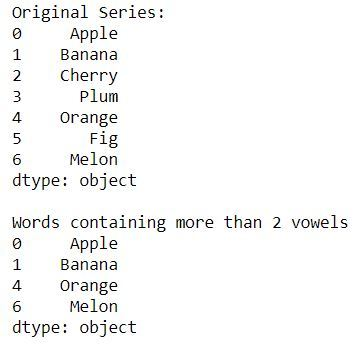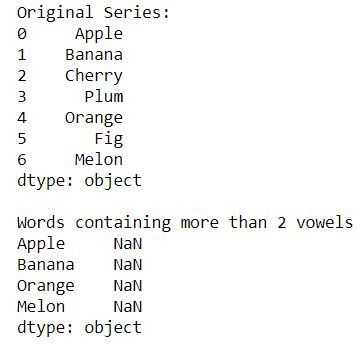Open in App
Not now

# Filter words from a given Pandas series that contain atleast two vowels

• Difficulty Level : Hard
• Last Updated : 27 May, 2021

In this article, how we can filter the words from a given series which contain two or more vowels. Here we’ll see two ways in which we can achieve this.
Example 1:
In this example we’ll use the map() function to loop through the series and check for each word if the count of vowels is greater than or equal to two. map is basically used to loop through the series and Counter is used to count the number of vowels in each word.

## Python3

 `import` `pandas as pd``from` `collections ``import` `Counter` `# creating a series of words``series ``=` `pd.Series([``'Apple'``, ``'Banana'``, ``'Cherry'``,``                    ``'Plum'``, ``'Orange'``, ``'Fig'``, ``'Melon'``])` `print``(``"Original Series:"``)``print``(series)``print``(``"\nWords containing atleast 2 vowels"``)` `# mapping through the series and checking if count of vowels is >=2``result ``=` `series.``map``(``lambda` `c: ``sum``([Counter(c.lower()).get(i, ``0``)``                                   ``for` `i ``in` `list``(``'aeiou'``)]) >``=` `2``)` `print``(series[result])`

Output:Example 2:
In this example we’ll use the Series.str.count() function with regex to loop through the series and check for each word if the count of vowels is greater than or equal to two. (?i) is used to start case-insensitive mode, to convert the uppercase character to lowercase characters. We need to convert uppercase characters to lowercase because for vowels we are comparing with lowercase vowels, so whenever an uppercase vowels appears like in Apple we need to convert ‘A’ to lowercase for proper comparison.

## Python3

 `import` `pandas as pd``from` `collections ``import` `Counter` `# creating a series of words``series ``=` `pd.Series([``'Apple'``, ``'Banana'``, ``'Cherry'``,``                    ``'Plum'``, ``'Orange'``, ``'Fig'``, ``'Melon'``])` `print``(``"Original Series:"``)``print``(series)``print``(``"\nWords containing atleast 2 vowels"``)` `# mapping through the series and checking``# if count of vowels is >=2``result ``=` `series[series.``str``.count(``'(?i)[aeiou]'``) >``=``2``]` `print``(series[result])`

Output:My Personal Notes arrow_drop_up